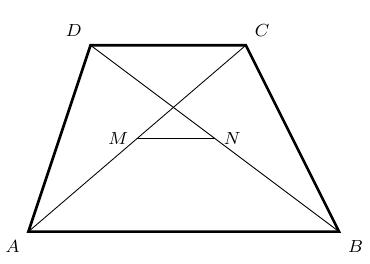VectorMethod Intermediate

Problem - 3104

Let $ABCD$ be a trapezoid. Points $M$ and $N$ are the mid points of its diagonal $AC$ and $BD$, respectively. Show that $MN \parallel AB$ and $MN = \frac{1}{2}\mid AB - CD\mid$.The solution for this problem is available for $0.99. You can also purchase a pass for all available solutions for$99.